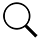Casino Player Magazine | Strictly Slots Magazine | Casino Gambling Tips# MIND GAMES

## What exactly does “random” mean, anyway?

### By Jerry “Stickman” Stich

In articles and books about video poker, we read about the odds of particular hands being made, and the house edge of various games. Discussions of odds or house edge are always predicated on a random game. For example, the odds of getting a four of a kind in full pay Jacks or Better are about 1 in 423 hands, in a random game.

But what does this mean, exactly?

Well it doesn’t mean that after 422 hands have been played that a four of a kind is next. It doesn’t even mean that after 800 hands or 1,200 hands or even 1,600 hands that a four of a kind is “due.” The whole concept of random is that the player cannot predict the outcome of the next hand.

But the human mind hates the idea of something being completely “random.” In fact, our brains despise it. Because of this, we subconsciously try to see patterns where none exist.Consider the series of digits in Table 1.

Notice how certain numbers are predominant at certain times and others are predominant at a different time. At one point in that series, there are six 9s in a row. Surely this is not random. There are patterns to the numbers. There is a “flow” of numbers from high to low, and back to high.

In actuality, the number series is 990 digits of the value of pi starting with the fourth digit after the decimal point. π (sometimes written pi) is a mathematical constant whose value is the ratio of any circle’s circumference to its diameter. This is the same value as the ratio of a circle’s area to the square of its radius. Pi is approximately equal to 3.14159 in the usual decimal positional notation.

Pi is an irrational number, which means that its value cannot be expressed exactly as a fraction n/d, where n (numerator) and d (denominator) are integers. Consequently, its decimal representation never ends or repeats. Pi is also a transcendental number, which implies, among other things, that no finite sequence of algebraic operations on integers (powers, roots, sums, etc.) can be equal to its value.

As of Aug. 5, 2010, the record for calculating the most digits of the value of pi was set at five trillion digits, without any series repeating. Now, five trillion may not be much compared to our national debt, but in terms of number series it is astronomical. Because of this, the value of pi can be considered random.

All the patterns that are noticed randomly occurred. They cannot be predicted for the future. If you counted all the occurrences of each digit in the five trillion digits of the value of pi, there would be approximately 500 billion of each digit. Just like randomness dictates that in the long run (and five trillion can certainly be considered as at least approaching the long run) you will see a four of a kind once in every 430 hands played, the player has no idea how long between the last four of a kind and the next. All that is certain is that the longer you play the more closely your results will come to the mathematical odds.

Now let me get to my point. When playing video poker, you may feel that you are “due” at times for a certain card or hand. But in reality, this is never the case. You have exactly the same odds of getting a particular hand on the first hand played, or the 50th hand played, or the 500th hand played…or even, should someone live long enough, the five trillionth hand played.

Random is exactly that. It is random. You cannot predict what is coming next.

Scroll to Top